## PyData Montreal slides for the talk: PyTorch under the hood

These are the slides of the talk I presented on PyData Montreal on Feb 25th. It was a pleasure to meet you all ! Thanks a lot to Maria and Alexander for the invitation !

## 10 years of blogging !

Hi all ! This post is to celebrate 10 years of blogging with an average of 1 post per month. It was a quite cool adventure !

Here is the full table of contents for those interested:

## A sane introduction to maximum likelihood estimation (MLE) and maximum a posteriori (MAP)

It is frustrating to learn about principles such as maximum likelihood estimation (MLE), maximum a posteriori (MAP) and Bayesian inference in general. The main reason behind this difficulty, in my opinion, is that many tutorials assume previous knowledge, use implicit or inconsistent notation, or are even addressing a completely different concept, thus overloading these principles.

Those aforementioned issues make it very confusing for newcomers to understand these concepts, and I’m often confronted by people who were unfortunately misled by many tutorials. For that reason, I decided to write a sane introduction to these concepts and elaborate more on their relationships and hidden interactions while trying to explain every step of formulations. I hope to bring something new to help people understand these principles.

## Maximum Likelihood Estimation

The maximum likelihood estimation is a method or principle used to estimate the parameter or parameters of a model given observation or observations. Maximum likelihood estimation is also abbreviated as MLE, and it is also known as the method of maximum likelihood. From this name, you probably already understood that this principle works by maximizing the likelihood, therefore, the key to understand the maximum likelihood estimation is to first understand what is a likelihood and why someone would want to maximize it in order to estimate model parameters.

Let’s start with the definition of the likelihood function for continuous case:

$$\mathcal{L}(\theta | x) = p_{\theta}(x)$$

The left term means “the likelihood of the parameters $$\theta$$, given data $$x$$”. Now, what does that mean ? It means that in the continuous case, the likelihood of the model $$p_{\theta}(x)$$ with the parametrization $$\theta$$ and data $$x$$ is the probability density function (pdf) of the model with that particular parametrization.

Although this is the most used likelihood representation, you should pay attention that the notation $$\mathcal{L}(\cdot | \cdot)$$ in this case doesn’t mean the same as the conditional notation, so be careful with this overload, because it is always implicitly stated and it is also often a source of confusion. Another representation of the likelihood that is often used is $$\mathcal{L}(x; \theta)$$, which is better in the sense that it makes it clear that it’s not a conditional, however, it makes it look like the likelihood is a function of the data and not of the parameters.

The model $$p_{\theta}(x)$$ can be any distribution, and to make things concrete, let’s say that we are assuming that the data generating distribution is an univariate Gaussian distribution, which we define below:

\begin{align} p(x) & \sim \mathcal{N}(\mu, \sigma^2) \\ p(x; \mu, \sigma^2) & \sim \frac{1}{\sqrt{2\pi\sigma^2}} \exp{ \bigg[-\frac{1}{2}\bigg( \frac{x-\mu}{\sigma}\bigg)^2 \bigg] } \end{align}

If you plot this probability density function with different parametrizations, you’ll get something like the plots below, where the red distribution is the standard Gaussian $$p(x) \sim \mathcal{N}(0, 1.0)$$: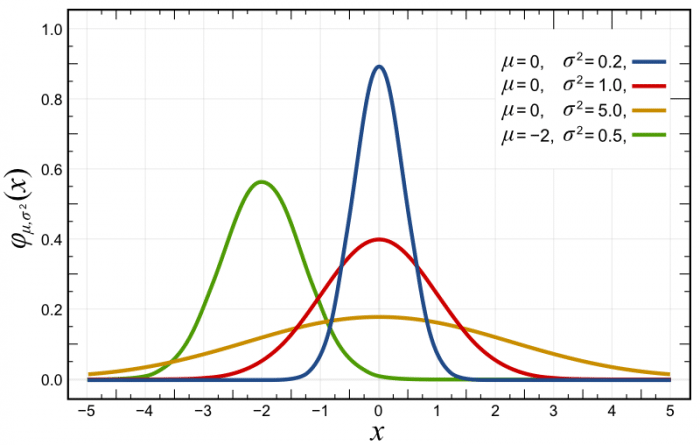A selection of Normal Distribution Probability Density Functions (PDFs). Both the mean, μ, and variance, σ², are varied. The key is given on the graph. Source: Wikimedia Commons.

As you can see in the probability density function (pdf) plot above, the likelihood of $$x$$ at variously given realizations are showed in the y-axis. Another source of confusion here is that usually, people take this as a probability, because they usually see these plots of normals and the likelihood is always below 1, however, the probability density function doesn’t give you probabilities but densities. The constraint on the pdf is that it must integrate to one:

$$\int_{-\infty}^{+\infty} f(x)dx = 1$$

So, it is completely normal to have densities larger than 1 in many points for many different distributions. Take for example the pdf for the Beta distribution below:Probability density function for the Beta distribution. Source: Wikimedia Commons.

As you can see, the pdf shows densities above one in many parametrizations of the distribution, while still integrating into 1 and following the second axiom of probability: the unit measure.

So, returning to our original principle of maximum likelihood estimation, what we want is to maximize the likelihood $$\mathcal{L}(\theta | x)$$ for our observed data. What this means in practical terms is that we want to find the parameters $$\theta$$ of our model where the likelihood that this model generated our data is maximized, we want to find which parameters of this model are most plausible to have generated this observed data, or what are the parameters that make this sample most probable ?

For the case of our univariate Gaussian model, what we want is to find the parameters $$\mu$$ and $$\sigma^2$$, which for convenient notation we collapse into a single parameter vector:

$$\theta = \begin{bmatrix}\mu \\ \sigma^2\end{bmatrix}$$

Because these are the statistics that completely define our univariate Gaussian model. So let’s formulate the problem of the maximum likelihood estimation:

\begin{align} \hat{\theta} &= \mathrm{arg}\max_\theta \mathcal{L}(\theta | x) \\ &= \mathrm{arg}\max_\theta p_{\theta}(x) \end{align}

This says that we want to obtain the maximum likelihood estimate $$\hat{\theta}$$ that approximates $$p_{\theta}(x)$$ to a underlying “true” distribution $$p_{\theta^*}(x)$$ by maximizing the likelihood of the parameters $$\theta$$ given data $$x$$. You shouldn’t confuse a maximum likelihood estimate $$\hat{\theta}(x)$$ which is a realization of the maximum likelihood estimator for the data $$x$$, with the maximum likelihood estimator $$\hat{\theta}$$, so pay attention to disambiguate it in your head.

However, we need to incorporate multiple observations in this formulation, and by adding multiple observations we end up with a complex joint distribution:

$$\hat{\theta} = \mathrm{arg}\max_\theta p_{\theta}(x_1, x_2, \ldots, x_n)$$

That needs to take into account the interactions between all observations. And here is where we make a strong assumption: we state that the observations are independent. Independent random variables mean that the following holds:

$$p_{\theta}(x_1, x_2, \ldots, x_n) = \prod_{i=1}^{n} p_{\theta}(x_i)$$

Which means that since $$x_1, x_2, \ldots, x_n$$ don’t contain information about each other, we can write the joint probability as a product of their marginals.

Another assumption that is made, is that these random variables are identically distributed, which means that they came from the same generating distribution, which allows us to model it with the same distribution parametrization.

Given these two assumptions, which are also known as IID (independently and identically distributed), we can formulate our maximum likelihood estimation problem as:

$$\hat{\theta} = \mathrm{arg}\max_\theta \prod_{i=1}^{n} p_{\theta}(x_i)$$

Note that MLE doesn’t require you to make these assumptions, however, many problems will appear if you don’t to it, such as different distributions for each sample or having to deal with joint probabilities.

Given that in many cases these densities that we multiply can be very small, multiplying one by the other in the product that we have above we can end up with very small values. Here is where the logarithm function makes its way to the likelihood. The log function is a strictly monotonically increasing function, that preserves the location of the extrema and has a very nice property:

$$\log ab = \log a + \log b$$

Where the logarithm of a product is the sum of the logarithms, which is very convenient for us, so we’ll apply the logarithm to the likelihood to maximize what is called the log-likelihood:

\begin{align} \hat{\theta} &= \mathrm{arg}\max_\theta \prod_{i=1}^{n} p_{\theta}(x_i) \\ &= \mathrm{arg}\max_\theta \sum_{i=1}^{n} \log p_{\theta}(x_i) \\ \end{align}

As you can see, we went from a product to a summation, which is much more convenient. Another reason for the application of the logarithm is that we often take the derivative and solve it for the parameters, therefore is much easier to work with a summation than a multiplication.

We can also conveniently average the log-likelihood (given that we’re just including a multiplication by a constant):

\begin{align} \hat{\theta} &= \mathrm{arg}\max_\theta \sum_{i=1}^{n} \log p_{\theta}(x_i) \\ &= \mathrm{arg}\max_\theta \frac{1}{n} \sum_{i=1}^{n} \log p_{\theta}(x_i) \\ \end{align}

This is also convenient because it will take out the dependency on the number of observations. We also know, that through the law of large numbers, the following holds as $$n\to\infty$$:

$$\frac{1}{n} \sum_{i=1}^{n} \log \, p_{\theta}(x_i) \approx \mathbb{E}_{x \sim p_{\theta^*}(x)}\left[\log \, p_{\theta}(x) \right]$$

As you can see, we’re approximating the expectation with the empirical expectation defined by our dataset $$\{x_i\}_{i=1}^{n}$$. This is an important point and it is usually implictly assumed.

The weak law of large numbers can be bounded using a Chebyshev bound, and if you are interested in concentration inequalities, I’ve made an article about them here where I discuss the Chebyshev bound.

To finish our formulation, given that we usually minimize objectives, we can formulate the same maximum likelihood estimation as the minimization of the negative of the log-likelihood:

$$\hat{\theta} = \mathrm{arg}\min_\theta -\mathbb{E}_{x \sim p_{\theta^*}(x)}\left[\log \, p_{\theta}(x) \right]$$

Which is exactly the same thing with just the negation turn the maximization problem into a minimization problem.

### The relation of maximum likelihood estimation with the Kullback–Leibler divergence from information theory

It is well-known that maximizing the likelihood is the same as minimizing the Kullback-Leibler divergence, also known as the KL divergence. Which is very interesting because it links a measure from information theory with the maximum likelihood principle.

The KL divergence is defined as:

$$\begin{equation} D_{KL}( p || q)=\int p(x)\log\frac{p(x)}{q(x)} \ dx \end{equation}$$

There are many intuitions to understand the KL divergence, I personally like the perspective on the likelihood ratios, however, there are plenty of materials about it that you can easily find and it’s out of the scope of this introduction.

The KL divergence is basically the expectation of the log-likelihood ratio under the $$p(x)$$ distribution. What we’re doing below is just rephrasing it by using some identities and properties of the expectation:

\begin{align} D_{KL}[p_{\theta^*}(x) \, \Vert \, p_\theta(x)] &= \mathbb{E}_{x \sim p_{\theta^*}(x)}\left[\log \frac{p_{\theta^*}(x)}{p_\theta(x)} \right] \\ \label{eq:logquotient} &= \mathbb{E}_{x \sim p_{\theta^*}(x)}\left[\log \,p_{\theta^*}(x) – \log \, p_\theta(x) \right] \\ \label{eq:linearization} &= \mathbb{E}_{x \sim p_{\theta^*}(x)} \underbrace{\left[\log \, p_{\theta^*}(x) \right]}_{\text{Entropy of } p_{\theta^*}(x)} – \underbrace{\mathbb{E}_{x \sim p_{\theta^*}(x)}\left[\log \, p_{\theta}(x) \right]}_{\text{Negative of log-likelihood}} \end{align}

In the formulation above, we’re first using the fact that the logarithm of a quotient is equal to the difference of the logs of the numerator and denominator (equation $$\ref{eq:logquotient}$$). After that we use the linearization of the expectation(equation $$\ref{eq:linearization}$$), which tells us that $$\mathbb{E}\left[X + Y\right] = \mathbb{E}\left[X\right]+\mathbb{E}\left[Y\right]$$. In the end, we are left with two terms, the first one in the left is the entropy and the one in the right you can recognize as the negative of the log-likelihood that we saw earlier.

If we want to minimize the KL divergence for the $$\theta$$, we can ignore the first term, since it doesn’t depend of $$\theta$$ in any way, and in the end we have exactly the same maximum likelihood formulation that we saw before:

$$\begin{eqnarray} \require{cancel} \theta^* &=& \mathrm{arg}\min_\theta \cancel{\mathbb{E}_{x \sim p_{\theta^*}(x)} \left[\log \, p_{\theta^*}(x) \right]} – \mathbb{E}_{x \sim p_{\theta^*}(x)}\left[\log \, p_{\theta}(x) \right]\\ &=& \mathrm{arg}\min_\theta -\mathbb{E}_{x \sim p_{\theta^*}(x)}\left[\log \, p_{\theta}(x) \right] \end{eqnarray}$$

### The conditional log-likelihood

A very common scenario in Machine Learning is supervised learning, where we have data points $$x_n$$ and their labels $$y_n$$ building up our dataset $$D = \{ (x_1, y_1), (x_2, y_2), \ldots, (x_n, y_n) \}$$, where we’re interested in estimating the conditional probability of $$\textbf{y}$$ given $$\textbf{x}$$, or more precisely $$P_{\theta}(Y | X)$$.

To extend the maximum likelihood principle to the conditional case, we just have to write it as:

$$\hat{\theta} = \mathrm{arg}\min_\theta -\mathbb{E}_{x \sim p_{\theta^*}(y | x)}\left[\log \, p_{\theta}(y | x) \right]$$

And then it can be easily generalized to formulate the linear regression:

$$p_{\theta}(y | x) \sim \mathcal{N}(x^T \theta, \sigma^2) \\ p_{\theta}(y | x) = -n \log \sigma – \frac{n}{2} \log{2\pi} – \sum_{i=1}^{n}{\frac{\| x_i^T \theta – y_i \|}{2\sigma^2}}$$

In that case, you can see that we end up with a sum of squared errors that will have the same location of the optimum of the mean squared error (MSE). So you can see that minimizing the MSE is equivalent of maximizing the likelihood for a Gaussian model.

### Remarks on the maximum likelihood

The maximum likelihood estimation has very interesting properties but it gives us only point estimates, and this means that we cannot reason on the distribution of these estimates. In contrast, Bayesian inference can give us a full distribution over parameters, and therefore will allow us to reason about the posterior distribution.

I’ll write more about Bayesian inference and sampling methods such as the ones from the Markov Chain Monte Carlo (MCMC) family, but I’ll leave this for another article, right now I’ll continue showing the relationship of the maximum likelihood estimator with the maximum a posteriori (MAP) estimator.

## Maximum a posteriori

Although the maximum a posteriori, also known as MAP, also provides us with a point estimate, it is a Bayesian concept that incorporates a prior over the parameters. We’ll also see that the MAP has a strong connection with the regularized MLE estimation.

We know from the Bayes rule that we can get the posterior from the product of the likelihood and the prior, normalized by the evidence:

\begin{align} p(\theta \vert x) &= \frac{p_{\theta}(x) p(\theta)}{p(x)} \\ \label{eq:proport} &\propto p_{\theta}(x) p(\theta) \end{align}

In the equation $$\ref{eq:proport}$$, since we’re worried about optimization, we cancel the normalizing evidence $$p(x)$$ and stay with a proportional posterior, which is very convenient because the marginalization of $$p(x)$$ involves integration and is intractable for many cases.

\begin{align} \theta_{MAP} &= \mathop{\rm arg\,max}\limits_{\theta} p_{\theta}(x) p(\theta) \\ &= \mathop{\rm arg\,max}\limits_{\theta} \prod_{i=1}^{n} p_{\theta}(x_i) p(\theta) \\ &= \mathop{\rm arg\,max}\limits_{\theta} \sum_{i=1}^{n} \underbrace{\log p_{\theta}(x_i)}_{\text{Log likelihood}} \underbrace{p(\theta)}_{\text{Prior}} \end{align}

In this formulation above, we just followed the same steps as described earlier for the maximum likelihood estimator, we assume independence and an identical distributional setting, followed later by the logarithm application to switch from a product to a summation. As you can see in the final formulation, this is equivalent as the maximum likelihood estimation multiplied by the prior term.

We can also easily recover the exact maximum likelihood estimator by using a uniform prior $$p(\theta) \sim \textbf{U}(\cdot, \cdot)$$. This means that every possible value of $$\theta$$ will be equally weighted, meaning that it’s just a constant multiplication:

\begin{align} \theta_{MAP} &= \mathop{\rm arg\,max}\limits_{\theta} \sum_i \log p_{\theta}(x_i) p(\theta) \\ &= \mathop{\rm arg\,max}\limits_{\theta} \sum_i \log p_{\theta}(x_i) \, \text{constant} \\ &= \underbrace{\mathop{\rm arg\,max}\limits_{\theta} \sum_i \log p_{\theta}(x_i)}_{\text{Equivalent to maximum likelihood estimation (MLE)}} \\ \end{align}

And there you are, the MAP with a uniform prior is equivalent to MLE. It is also easy to show that a Gaussian prior can recover the L2 regularized MLE. Which is quite interesting, given that it can provide insights and a new perspective on the regularization terms that we usually use.

I hope you liked this article ! The next one will be about Bayesian inference with posterior sampling, where we’ll show how we can reason about the posterior distribution and not only on point estimates as seen in MAP and MLE.

– Christian S. Perone

## Introducing EuclidesDB – A machine learning feature databasePast week I released the first public version of EuclidesDB. EuclidesDB is a multi-model machine learning feature database that is tightly coupled with PyTorch and provides a backend for including and querying data on the model feature space.

For more information, see the GitHub repository or the documentation.

Some features of EuclidesDB are listed below:

• Written in C++ for performance;
• Uses protobuf for data serialization;
• Uses gRPC for communication;
• LevelDB integration for database serialization;
• Many indexing methods implemented (AnnoyFaiss, etc);
• Tight PyTorch integration through libtorch;
• Easy integration for new custom fine-tuned models;
• Easy client language binding generation;
• Free and open-source with permissive license;

And here is a diagram of the overall architecture: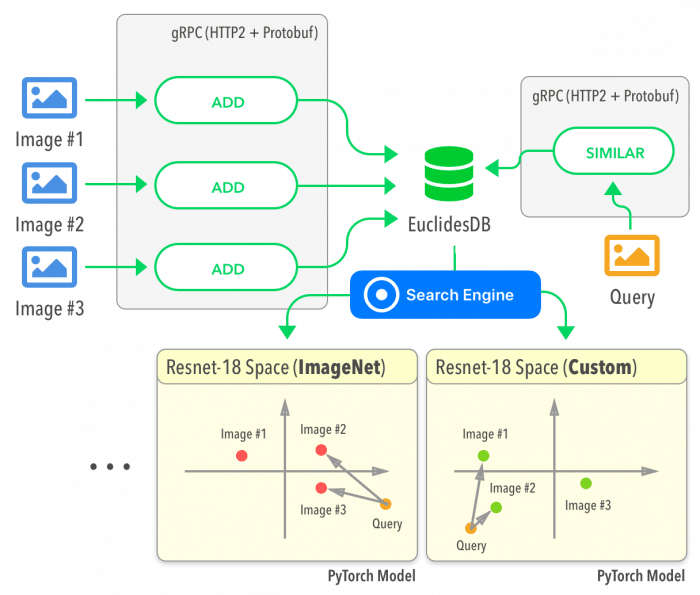## Time Maps: visualizing discrete events from Brazilian presidential election candidates

### Introduction

This last presidential election in Brazil was heavily marked by huge amounts of money being funneled to digital agencies and all kinds of targeting businesses that used Twitter, WhatsApp, and even SMS messages to propagate their content using their targeting strategies. Even before the elections, Cambridge Analytica was recorded mentioning their involvement in Brazil.

What makes Brazil so vulnerable for these micro-targeting companies, in my opinion, is the widespread ingenuity regarding digital platforms. An example of this ingenuity was the wide-spreading of applications that were allegedly developed to monitor politicians and give information about them, to help you decide your vote, bookmark politicians, etc. But in reality, it was more than clear that these applications were just capturing data (such as geolocation, personal opinions, demographics, etc) about their users with the intention to sell it later or use themselves for targeting. I even saw journalists and some very well-known people supporting these applications. Simply put, most of the time, when you don’t pay for a product (or application), you’re the product.

One very interesting work is the experiment done by Wu Youyou in 2014 where he showed that a simple regularized linear model was better or equal in accuracy to identify some personality traits using Facebook likes, this study used more than 80k participants data: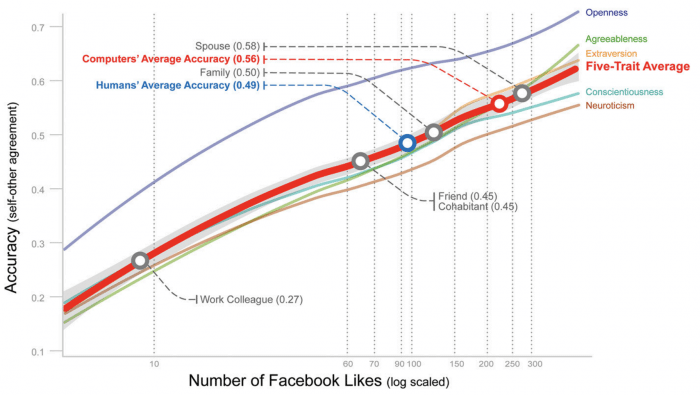Figure from: “Computer-based personality judgments are moreaccurate than those made by humans”. By Wu Youyou et al.

This graph above shows that with 70 likes from your Facebook, the linear model was more accurate than the evaluation of a friend of you and with more than 150 likes it can reach the accuracy of the evaluation of your family. Now you can understand why social data is so important for these companies to identify personality traits and content that you’re most susceptible.

### Time Maps

In this year, one of the candidates didn’t participate much on the debates before the second round and used mostly digital platforms to reach voters, so Twitter became a very important medium that all candidates explored in some way. The idea of this post is to use a discrete event visualization technique called Time Maps which was extended to Twitter visualizations by Max C. Watson in his work “Time Maps: A Tool for Visualizing Many Discrete Events Across Multiple Timescales” (paper available here). It is unfortunate that not a lot of people use these visualizations because they are very interesting for the visualization of activity patterns in multiple time-scales on a single plot.

The main idea behind time maps is that you can visualize the time after and before the events for the entire discrete time events. This can be easily understood by looking at the visual explanations done by Max C. Watson.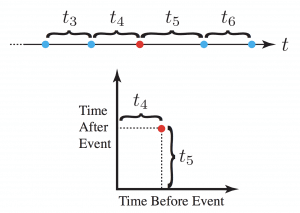Image from: Time Maps: A Tool for Visualizing Many Discrete Events Across Multiple Timescales. By Max C. Watson.

As you can see in the right, the plot is pretty straightforward, it might take some time for you to realize what is the meaning of the x and y-axes, but once you grasp the concept, you’ll see that they are quite easy to interpret and how many patterns it can reveal on a single plot.

Time maps were an adaptation from the chaotic field where they were initially developed to study the timing of water drops.

One way to easily understand it is to look at these two series below and their respective time maps:Image from: Time Maps: A Tool for Visualizing Many Discrete Events Across Multiple Timescales. By Max C. Watson.

But before plotting the time maps, let’s explore some basic visualizations from the two candidates who got into the second round of the general elections last week.

### Basic visualizations

I’ll focus only on the two candidates who got into the second round of the elections, their names are Jair Bolsonaro (president elected) and Fernando Haddad (not elected). These first plots will show the number of tweets per day during the year of 2018 and with some red marks indicating the first and second rounds of the elections: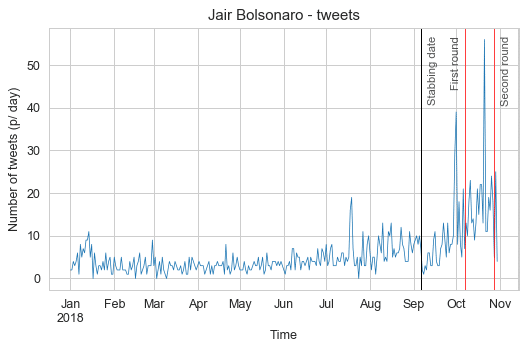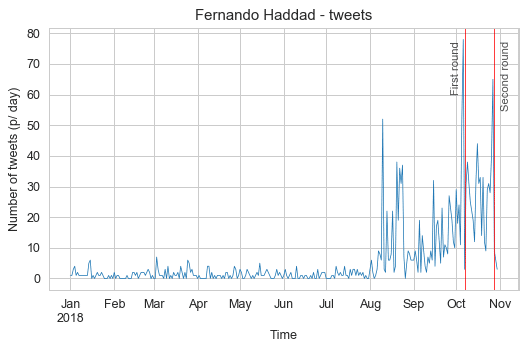In these plots, we can see that Jair Bolsonaro was more active before the general elections and that for both candidates, the aggregated number of tweets per day always peaked before each election round, with Jair Bolsonaro peaks happening a little earlier than Fernando Haddad. I also marked with a black vertical line the day that Jair Bolsonaro was stabbed in the streets of Brazil, you can see a clear drop of activity with a slow recovery after it.

Let’s now see the time of day profile for each candidate to check the hours of the day that the candidates were quieter and more active: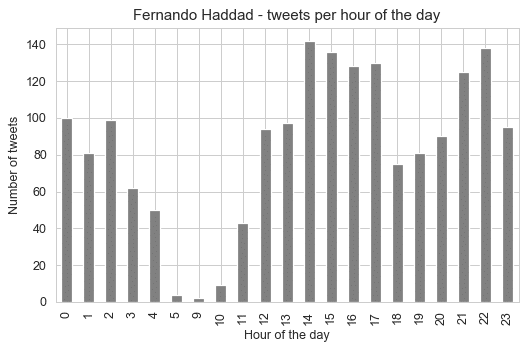These profiles tell us very interesting information, that the candidates were most active between 3pm and 4pm, but for Jair Bolsonaro, it seems that the 3pm time of the day is really the time when he was most active by a significant margin. What is really interesting is that there is no tweet whatsoever between 6am and 8am for Fernando Haddad.

Let’s look now the distribution differences between 2017 and 2018 for each candidate:As we can see from these plots, Jair Bolsonaro was as active in 2017 as in 2018, while Fernando Haddad was not so much active in 2017 with a huge bump in a number of tweets in the year of 2018 (election year). Something that is interesting, is that the pattern from Jair Bolsonaro to tweet more at 1pm shifted to 3pm in 2018, while for Haddad it changed also from 1pm to 2pm. It can be hypothesized that before they were less involved and used to tweet after lunch, but during election year this routine changed (assuming that it’s not their staff who is managing the account for them), so there is not only more tweets but also a distributional shift in the hour of the day.

### Time Map Visualization

These are the time maps for Jair Bolsonaro. The first is the time map colored by the hour of the day and the second time map is a heat map to see the density of points in the time map.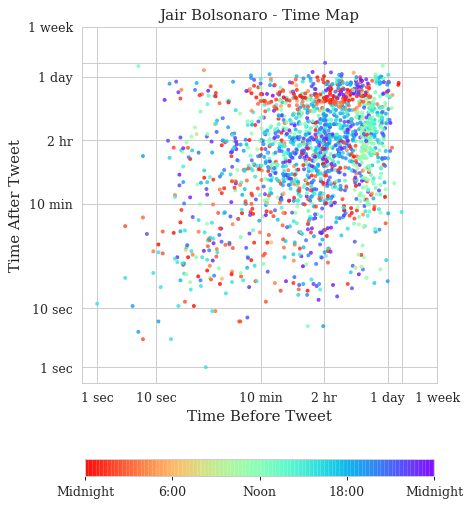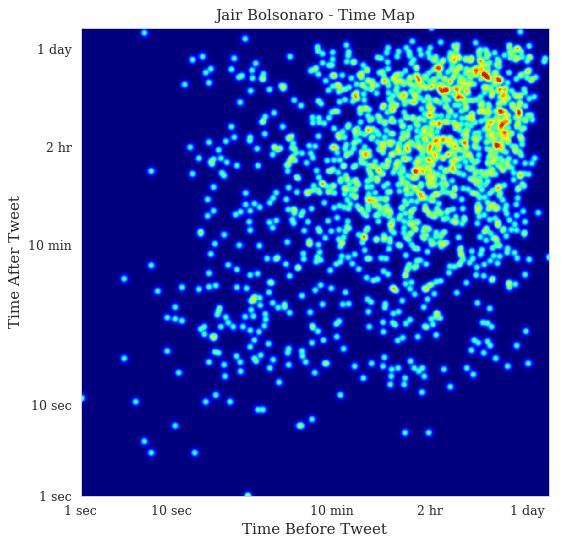And these are the time maps for Fernando Haddad: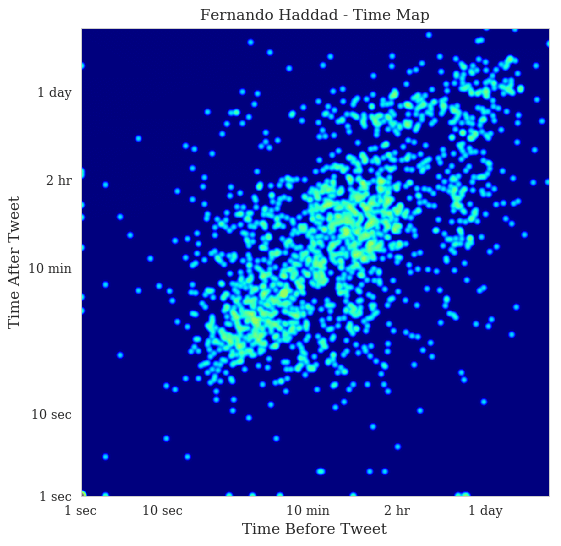Now, these are very interesting time maps. You can clearly see in the Jair Bolsonaro time map that there are two stripes: vertical on the left and horizontal on the top that shows the first and last tweets of the day respectively. It’s a slow but steady activity of tweeting, with a concentration on the heat map on the 1-day bands. In Fernando Haddad, you can see that the stripes are still visible but much less concentrated. There are also two main blobs in the heat map of Fernando Haddad, one in the bottom left showing fast tweets that are probably from a specific event and then the blob on the top right showing the usual activity.

If you are interested in understanding more about these plots, please take a look on Max Watson blog article where he explains some interesting cases such as the tweets from the White House account.

### Spotting bots with Time Maps

If you are curious about how Twitter bots appear on time maps, here is an example where I plot the tweets from the CozinhaBot, that keeps posting some random recipes on twitter: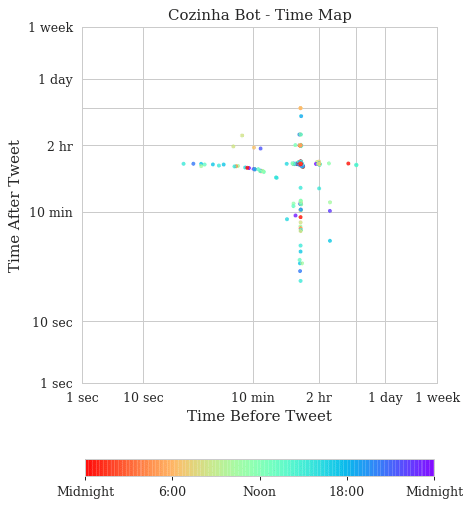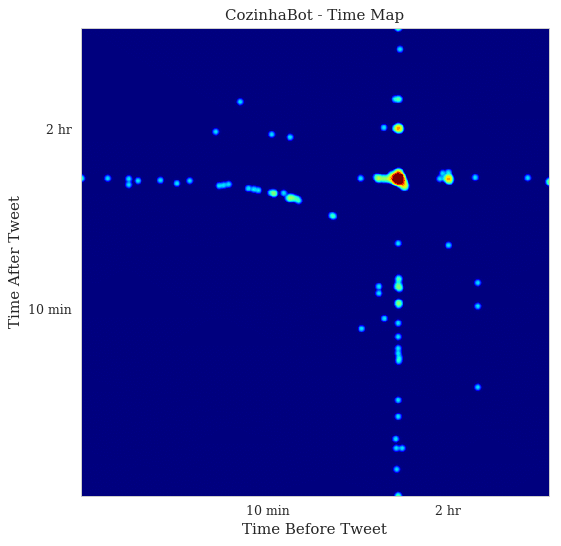As you can see, the pattern is very regular, in the heat map we can see the huge density spot before the 2 hr ticks, which means that this bot has a very well known and regular pattern, as opposed to the human-produced patterns we saw before. These plots don’t have a small amount of dots because it has fewer tweets, but because they follow a very regular interval, this plot contains nearly the same amount of tweets we saw from the previous examples of the presidential candidates. This is very interesting because not only can be used to spot twitter bots but also to identify which tweets were posted out of the bot pattern.

I hope you liked !

– Christian S. Perone

## Análise bayesiana dos microdados do ENEM do Rio Grande do Sul

* This post is in Portuguese. It’s a bayesian analysis of a Brazilian national exam. The main focus of the analysis is to understand the underlying factors impacting the participants performance on ENEM.

Este tutorial apresenta uma análise breve dos microdados do ENEM do Rio Grande do Sul do ano de 2017. O principal objetivo é entender os fatores que impactam na performance dos participantes do ENEM dado fatores como renda familiar e tipo de escola. Neste tutorial são apresentados dois modelos: regressão linear e regressão linear hierárquica.